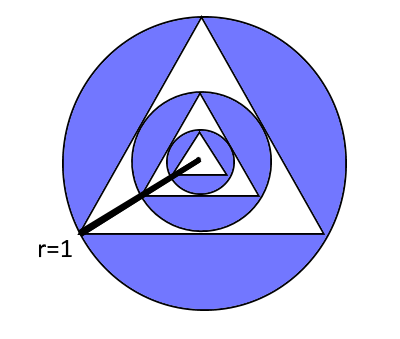# Endless Circles and Triangles

Geometry Level 3

An equilateral triangle is drawn inside a circle of radius 1, such that all vertices of the triangle touch the circle.
Another circle is drawn inside the triangle such that the new circle touches each side of the triangle once.
Another equilateral triangle is drawn inside the second circle as described above, and a third circle is drawn inside the second triangle as described above.
This process is continued to form an infinite number of circles and triangles.
The first three circles and triangles are shown in the picture below.What is the ratio (in percentage) of the ​area of the blue region to the area of the largest circle?